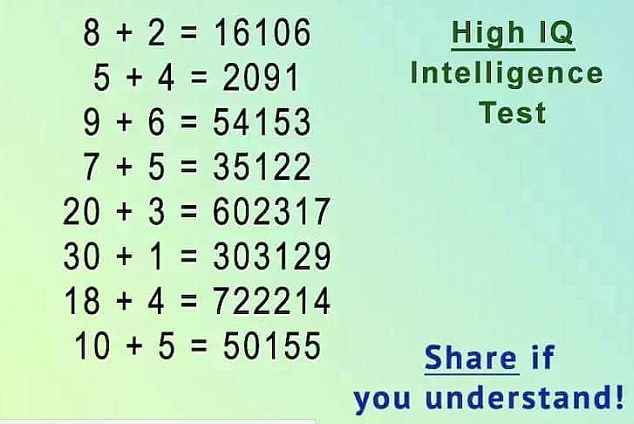# Can You Crack This Brain Teaser?Published1Comment

Once again, a brain teaser is confusing the hell out of people on the Internet. More than a hundred thousand people shared this one this week!

This time around it's a mathematical puzzle. Don’t be fooled by the long numbers, though. You should be able to solve it with some basic math skills. All you need to do is work out the pattern that gives you the long number in each line.

If you’re still stumped, you can see how to solve it below the post.To work out the answer, all you need to do is multiply the two numbers, then add them together, then subtract them from each other. Then just put these three answers together.

So, for example, on the first line: 8 x 2 = 16, 8 + 2 = 10, 8-2 = 6. Put those numbers together in order and you’ll get 16106.

### ARTICLE POSTED IN

•• math,

• mathematics,

• puzzle,

• quiz,

• brain teaser